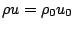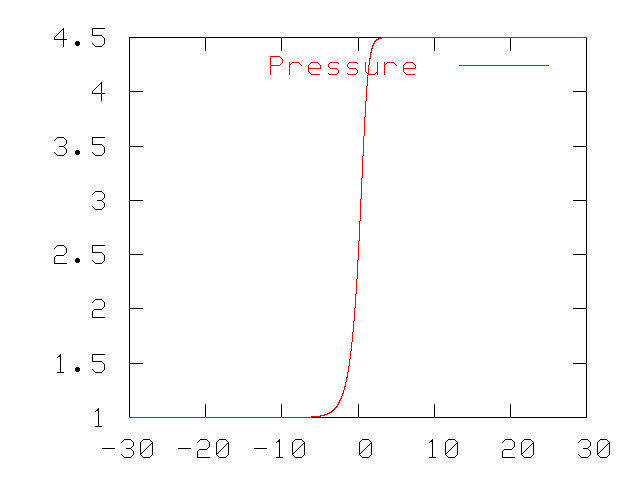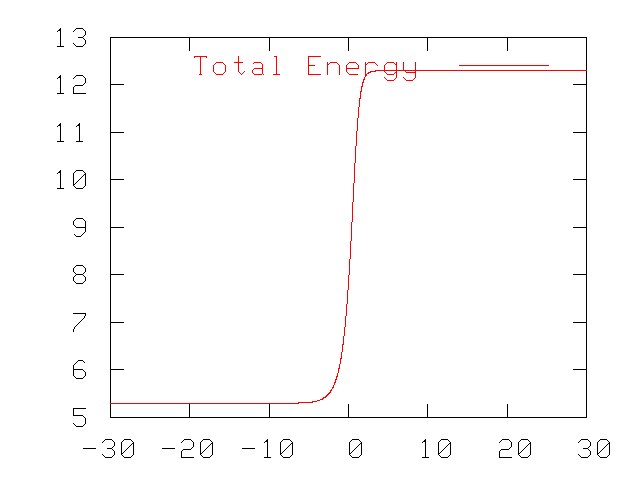Jump:

Start of topic | Skip to actions

# 1D Navier-Stokes equations - Stationary shock wave

## Problem description

The exact solution of a stationary shock wave for the one dimensional Navier-stokes equations has been used as an AMROC verification problem. In a shock stationary frame of reference, the 1D Navier-Stokes equations for constant viscosity and thermal conductivity arewhich is supplemented by the ideal gas law,This solution has a Prandtl number equal to 3/4, which changes the energy equation to an algebraic equation:using the constraints,with the following non dimensionalization,The analytical solution is formulated in non-dimensional form, with the upstream density, pressure, and velocity in addition to an equivalent perfect gas mean free path are used to non-dimensionalize the solution. The mean free path is quite abritrary, and for the solution used isAll the above simplifies the 3 PDE's of the 1D Navier-Stokes equations to one ODE, which is solved analytically. See Kramer et al for the implicit solution, which is expressed as a function of the Mach number and specific heat ratio and relates the non-dimensional velocity and position. The density, pressure, and hence the total energy are found with the relations,This derivation is carried out for for the monoatomic gas case,, with different arbitrary perfect gas equivalent mean free path in the textbook by Zel'dovich and Raizer.

### Initial / Boundary Conditions

This specific exact solution is available in the weno and clawpack: applications/euler/1D/ViscousShock directories. For the following tests, we use the computational domain, x = [-30,30], inflow/outflow boundary conditions and the parameterswhere the 0 subsript denotes the upstream flow with Mach number equal to two. The domain is large enough and the final time short enough, to minimize the boundary errors.

### CFL number definition:

By using this exact solution as a verification problem an equivalent CFL number was derived. "CFL number" in this context, is the Navier-Stokes CFL number (including both convective and diffusive operators and analogous to the Euler equations case) that is used in a CFL condition. This CFL condition garrantees a stable solution for which the CFL number is less than or equal to one.

### Results:

A stable solution and the order of accuracy for both Clawpack's 2nd order slope-limited Roe solvers and WENO was verified, with viscous and heat conduction terms for a perfect gas. In the process, the differences in the calculations of the CFL number were found in the new Navier-Stokes modification for Clawpack with that of the current WENO-TCD implementation. In actuality, the CFL number for stability of an Explicit method is very complicated for the full one and three dimensional Navier-Stokes equations. However, using a condition which is based on a one-dimensional advection-diffusion equation  (which virtually adds the convective, diffusive, and thermal conditions together rather than taking a maximum over all) is robust and stable up to CFL = 1, . The current WENO implementation is stable and non-ossillatory for this verification problem only up to CFL=~0.6, as it used a less robust CFL number.

The newly implemented CFL number evaluation for Clawpack in 1D iswhere the maximum is take over all cell centers. This is derived by using Von Neumann analysis and ignoring theterm.

 R.M.J. Kramer, C. Patano, D.I. Pullin. A class of energy stable, high-order finite-difference interface schemes suitable for adaptive mesh refinement of hyperbolic problems. J. Comput. Phys., 226:1458-1484, 2007.

 A.C. Hindmarsh, P.M. Gresho, and D.F. Griffiths. The stability of explicit Euler time-integration for certain finite difference approximations of the multi-dimensional advection-diffusion equation. Inter. J. Num. Methods in Fluids, 4:853-897, 1984.

 Y.B. Zel'dovich and Y.P. Raizer. Physics of shock waves and high-temperature hydrodynamic phenomena. Dover. 2002.-- JackZiegler? - 29 Jul 2008

I AttachmentSize Date Who Commentvelocity.ps 40.5 K 29 Jul 2008 - 12:54 JackZiegler? velocityvelocity.png 4.2 K 29 Jul 2008 - 13:12 JackZiegler? velocityentropy.gif 4.1 K 29 Jul 2008 - 13:19 JackZiegler? entropy

You are here: Amroc > ViscousShockWaveConvergenceStudy > ViscousShock

to top

Copyright © 1997-2019 California Institute of Technology.